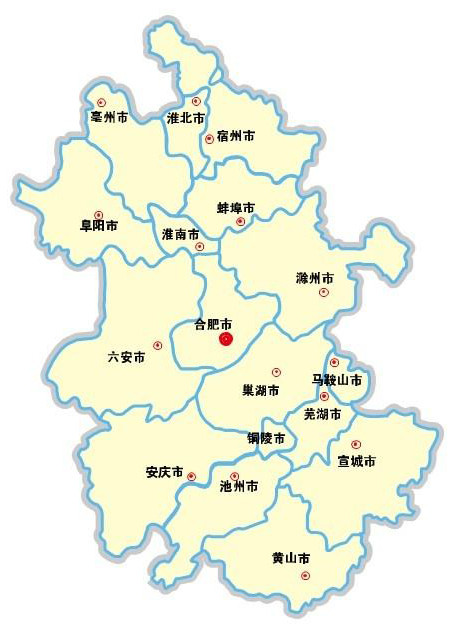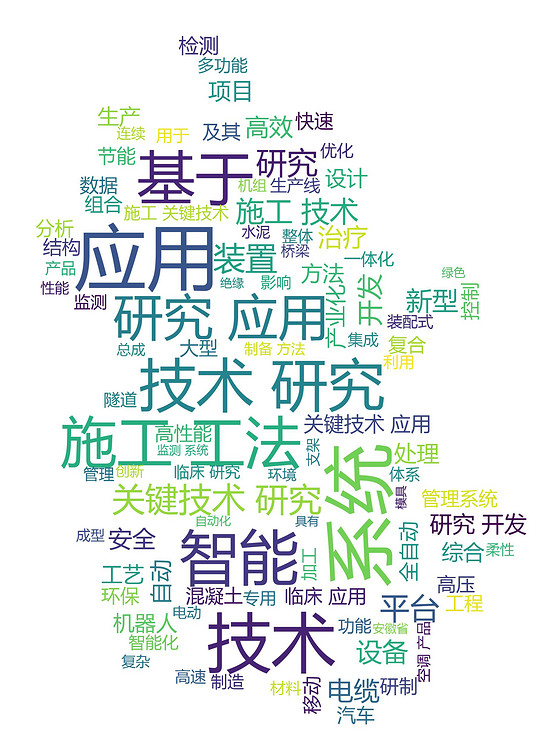# 用Python和WordCloud绘制词云的实现方法(内附让字体清晰的秘笈)

更新时间：2019年01月08日 14:46:59   作者：HateMath归来我要评论

• Win7 64位
• Python 3.6.4
• WordCloud 1.5.0
• Pillow 5.0.0
• Jieba 0.39

```import numpy as np
from PIL import Image
import re
import jieba
from wordcloud import WordCloud,ImageColorGenerator,STOPWORDS
import matplotlib.pyplot as plt
# http://www.cnblogs.com/hatemath/
# 打开存放项目名称的txt文件
with open('content.txt','r',encoding='utf-8') as f:
f.close()

# 图片模板和字体
image=np.array(Image.open('ditu.jpg'))
font=r'C:\\Windows\\fonts\\msyh.ttf'

# 去掉英文，保留中文
resultword=re.sub("[A-Za-z0-9\[\`\~\!\@\#\\$\^\&\*\(\)\=\|\{\}\'\:\;\'\,\[\]\.\<\>\/\?\~\。\@\#\\\&\*\%]", "",word)
wordlist_after_jieba = jieba.cut(resultword)
wl_space_split = " ".join(wordlist_after_jieba)

# 设置停用词
sw = set(STOPWORDS)

# 关键一步
max_words = 100,max_font_size = 60,random_state=20).generate(wl_space_split)

#显示生成的词云
plt.imshow(my_wordcloud)
plt.axis("off")
plt.show()

#保存生成的图片
my_wordcloud.to_file('result.jpg')``````# 关键一步